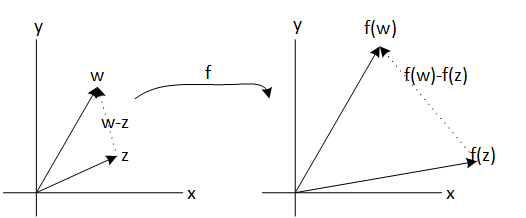# Complex derivate - Holomorphic functions

### Basic concepts and principles

As we will see, in complex case, derivative concept is much stronger than case of real variable functions. In this latter case, a function is differentiable at a point if partial derivatives exist and are continuous functions at that point.

The complex case is analogous, but it adds an extra condition: a system of partial differential equations called Cauchy - Riemann equations , must hold too.

### Cauchy - Riemann equations

Given a complex variable function: $$f: U \subset \mathbb{C} \mapsto \mathbb{C}$$ If complex derivate exists, f'(z) then Cauchy - Riemann equations , holds

$u_{x} = v_{y}$
$u_{y} = -v_{x}$

In such case it is said that f is Holomorphic

Complex derivate condition existence is very restrictive, for example f we take the conjugate function

$f(z) = \bar{z}$

Take real and imaginary parts of f

$u(x, y)= x,\: v(x,y) = -y$

Note that both functions have a good behavior (continuity, differentiability, ...), however, the function f has no complex derivative at any point because Cauchy - Riemann equations never holds in any point

The previous example shows, as said, that the existence condition of a complex derivative is much stronger than in the case of real variable functions. Anticipating the content of the following sections, when a function has a complex derivative at a point, then there are derivatives of all orders and therefore the function can be developed in series of powers around that point. In which case we will adopt the function term Holomorphic

We want to highlight that in many books, the analytical term appears to refer to a complex function that has derivative. All holomorphic function is analytical and reciprocally, all analytic function is Holomorphic, however, in complex variable a function with a single derivative is analytic, which does not occur in real variable, therefore, in my opinion the term holomorphic function is more appropriate for the particular case of a complex variable function.

### The complex derivate concept

Complex derivate f(x,y)= u(x,y) + iv(x,y) is defined as
$$f'(z)=\lim_{w \mapsto z } \frac{f(z)-f(w)}{z-w}$$Lets see that the complex derivative is to take the quotient between the distance between f(z) and f(w), the distance between z and w and then make the limit. This is the geometric interpretation of the complex derivative.

Note that if we take directional derivatives, for example if we approximate the real part, we take

$z=x+iy$
$w=x+h+iy$

Then

$f'(z)=\lim_{h \mapsto 0 } \frac{ u(x+h,y)+iv(x+h,y) - (u(x,y)+iv(x,y)) }{x+h+iy-(x+iy)} =$ $\frac{ u(x+h,y)-u(x,y) }{h} + i\frac{ v(x+h,y)-v(x,y) }{h} = u_{x} + i v_{x}$

On the other hand, if we approach by the imaginary part, that is, we take

$z=x+iy$
$w=x+i(y+h)$

Tenemos

$f'(z)=\lim_{h \mapsto 0 } \frac{ u(x,y+h)+iv(x,y+h) - (u(x,y)+iv(x,y)) }{x+i(y+h)-(x+iy)}$ $= \frac{ u(x,y+h)-u(x,y) }{ih} - i\frac{ v(x,y+h)-v(x,y) }{ih} = v_{y} - i u_{y}$

Note tha both force the condtion of Cauchy - Riemann equations

Cauchy - Riemann equations they are a necessary condition for the complex derivative, but they are not sufficient, the continuity of the partial derivatives of u and v must also be assumed. as we see in the following theorem

### Converse of Cauchy - Riemann equations

Given two functions u (x, y) and v (x, y), such that verify the Cauchy - Riemann equations and with continuous partial derivatives in an open set $U \subset \mathbb{C}$, then funcion:
$$f(x,y) = u(x,y) + iv(x,y)$$
has complex derivate $\forall z \in U$

Now, it suffices to observe that if the derivative of f '(z) could also be calculated, that is, the second derivative of f (z), then the Cauchy - Riemann equations for f'(z), ie $$f'(z) = u_{x} +iv_{x}$$ so, for Cauchy - Riemann equations applied to f' together with the equality of cross-sectional derivatives:
$$u_{xx} = v_{xy} = (-u_{y})_{y} = -u_{yy}$$ $$u_{xy} = - v_{xx} \Rightarrow -v_{xx} = (v_{y})_{y}$$
Then finally
$$u_{xx} + u_{yy} = 0$$ $$v_{xx} + v_{yy} = 0$$
In the other hand u(x,y) e v(x,y) are harmonic function.

This shows the following theorem

### Harmonic functions

Given a function of complex variable whose derivative exists in an open set $U \subset \mathbb{C}$. So the real part and the imaginary part are harmonic functions in $U \subset \mathbb{R}^{2}$, es decir $\forall x \in U$ it is holds $$u_{xx} + u_{yy} = 0$$ $$v_{xx} + v_{yy} = 0$$

Given a function of complex variable whose derivative exists in an open set $U \subset \mathbb{C}$. So the real part and the imaginary part are harmonic functions.

But there is still more, there is a kind of reciprocal that ensures that given a harmonic function u(x, y), then there is a harmonic conjugate v (x, y) such that

f(x,y) = u(x,y) + iv(x,y)

Is a Holomorphic function.

## Was useful? want add anything?

Post here

### Post from other users

Post here